# Multiple MCQ Of Moving Charges And Magnetism, Past Year Questions JEE Advance, Class 12, Physics

## 21 Questions MCQ Test Class 12 Physics 35 Years JEE Mains &Advance Past year Paper | Multiple MCQ Of Moving Charges And Magnetism, Past Year Questions JEE Advance, Class 12, Physics

Description
This mock test of Multiple MCQ Of Moving Charges And Magnetism, Past Year Questions JEE Advance, Class 12, Physics for JEE helps you for every JEE entrance exam. This contains 21 Multiple Choice Questions for JEE Multiple MCQ Of Moving Charges And Magnetism, Past Year Questions JEE Advance, Class 12, Physics (mcq) to study with solutions a complete question bank. The solved questions answers in this Multiple MCQ Of Moving Charges And Magnetism, Past Year Questions JEE Advance, Class 12, Physics quiz give you a good mix of easy questions and tough questions. JEE students definitely take this Multiple MCQ Of Moving Charges And Magnetism, Past Year Questions JEE Advance, Class 12, Physics exercise for a better result in the exam. You can find other Multiple MCQ Of Moving Charges And Magnetism, Past Year Questions JEE Advance, Class 12, Physics extra questions, long questions & short questions for JEE on EduRev as well by searching above.
*Multiple options can be correct
QUESTION: 1

### A magnetic needle is kept in a non uniform magnetic field. It experiences

Solution:

The force on north pole =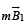The force on south pole =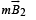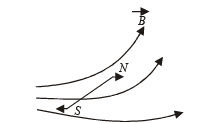Since the forces will be unequal and are not having same line of action therefore, the magnetic needle experiences a force as well as a torque.

*Multiple options can be correct
QUESTION: 2

### A proton moving with a constant velocity passes through a region of space without any change in its velocity. If E and B represent the electric and magnetic fields respectively, this region of space may have :

Solution:

There is no change in velocity. It can be possible when 

Electric and magnetic fields are absent, i.e., E = 0, B = 0 

Or when electric and magnetic fields are present but force due to electric field is equal and opposite to the magnetic force, (i .e., E ≠ 0, B ≠ 0). 

Or when E = 0. B ≠ 0 provided

F = qvB sin θ = 0

sin θ = 0, i.e., θ = 0 ⇒ v and B are in the same direction.

*Multiple options can be correct
QUESTION: 3

### A rectangular loop carrying a current i is situated near a long straight wire such that the wire is parallel to one of the sides of the loop and is in the plane of the loop. If steady current I is established in the wire as shown in the figure, the loop will :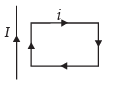Solution:

AB part of the rectangular loop will get attracted to the long straight wire as the currents are parallel and in the same direction whereas CD part will be repelled. But since this force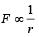where r is the distance between the wires. Therefore, there will be a net attractive force on the rectangular loop. Force on BC is equal and opposite to that on AD.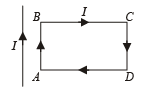*Multiple options can be correct
QUESTION: 4

Two thin long parallel wires seperated by a distance ‘b’ are carrying a current ‘i’ amp each. The magnitude of the force per unit lenght exerted by one wire on the other is

Solution:

The magnetic field due to current in wire 1 in the region of wire 2 will be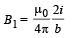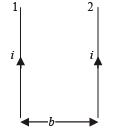Since wire 2 having current i is placed in a magnetic field B1, it will experience a force given by F = i (lB1 sin 90°)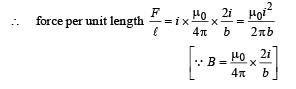*Multiple options can be correct
QUESTION: 5

Two particles X and Y having equal charges, after being accelerated through the same potential difference, enter a region of uniform magnetic field and describe circular paths of radii R1 and R2 respectively. The ratio of the mass of X to that of Y is

Solution:

K.E. of first particle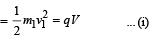K.E. of second particle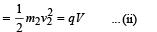NOTE : After entering the magnetic field, a magnetic force acts on the charged particle which moves the charged particle in circular path of radius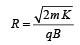Here, K, q, B are equal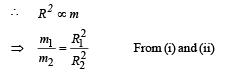*Multiple options can be correct
QUESTION: 6

A particle of charge + q and mass m moving under the influence of a uniform electric field Eiˆ and uniform magnetic field B kˆ follows a trajectory from P to Q as shown in fig. The velocities at P and Q are viˆ and – 2vˆj . Which of the following statement (s) is/are correct ?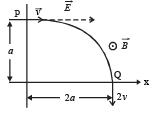Solution:

Considering the activity from P to Q (Horizontal) u1 = v, v1 = 2v, s1 = 2a, Acc = A

⇒ 4v2 – v2 = 2A(2a)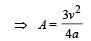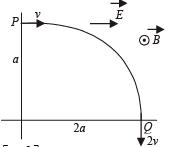Force acting in the horizontal direction is F = qE = mA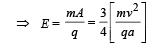Rate of doing work at P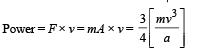Rate of doing work by the magnetic field is throughout zero.The rate of doing work by electric field is zero at Q. Because at Q, the angle between force due to electric field and displacement is zero.

*Multiple options can be correct
QUESTION: 7

A microameter has a resistance of 100 W and a full scale range of 50 mA . It can be used as a voltmeter or as a higher range ammeter provided a resistance is added to it. Pick the correct range and resistance combination (s)

Solution:

For V = Ig (G + R) = 5 × 10–5 [100 + 200,000] = 10V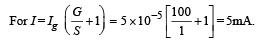*Multiple options can be correct
QUESTION: 8

A current I flows along the length of an infinitely long, straight, thin-walled pipe. Then

Solution:

Let us consider any poin t P inside the thin walled pipe. Let us consider a circular loop and apply Ampere's circuital law,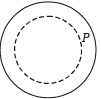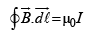Since current inside the loop is zero.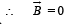*Multiple options can be correct
QUESTION: 9

H+, He+ and O++ all having the same kinetic energy pass through a region in which there is a uniform magnetic field perpendicular to their velocity. The masses of H+, He+ and O2+ are 1 amu, 4 amu and 16 amu respectively. Then

Solution:

KEY CONCEPT : When the charged particles enter a magnetic field then a force acts on the particle which will act as a centripetal force. We know that when kinetic energy and magnetic field are equal then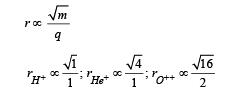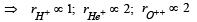He+ and O++ will be deflected equally.
H+ will be deflected the most since its radius is smallest.

*Multiple options can be correct
QUESTION: 10

Two particles, each of mass m and charge q, are attached to the two ends of a light rigid rod of length 2R. The rod is rotated at constant angular speed about a pependicular axis passing through its centre. The ratio of the magnitudes of the magnetic moment of the system and its angular momentum about the centre of the rod is

Solution: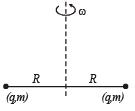Current, i = (frequency) (charge)=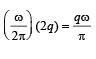Magnetic moment,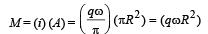Angular momentum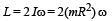.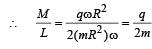*Multiple options can be correct
QUESTION: 11

Two very long, straight, parallel wires carry steady currents I & -I respectively. The distance between the wires is d. At a certain instant of time, a point charge q is at a point equidistant from the two wires, in the plane of the wires. Its instantaneous velocity v is perpendicular to this plane. The magnitude of the force due to the magnetic field acting on the charge at this instant is

Solution:

Net magnetic field due to the wires will be downward as shown below in the figure. Since angle between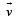and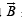is 180°,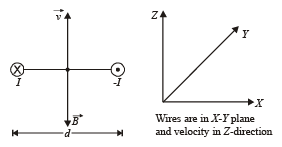Therefore, magnetic force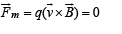*Multiple options can be correct
QUESTION: 12

The following field line can never represent

Solution:

Out of the given options only induced electric field and magnetostatic field form closed loops of field lines.

*Multiple options can be correct
QUESTION: 13

A long current carrying wire, carrying current I1 such that I1 is flowing out from the plane of paper is placed at O. A steady state current I2 is flowing in the loop ABCD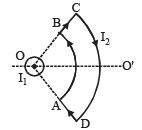Solution:

Net force on the loop : Force on AB : The magnetic field due to current I1 is along AB.

dF = I (d l * B ´ sin 0°)=0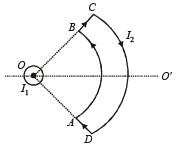Force on CD : Similarly the magnetic field due to current I1 is along DC. Because θ = 180° here, therefore force on DC is zero.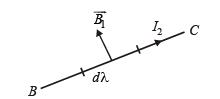Force on BC : Consider a small element dl.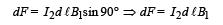By Fleming's left hand rule, the direction of this force is perpendicular to the plane of the paper directed outwards.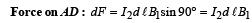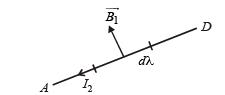By Fleming's left hand rule, the direction of this force is perpendicular to the plane of paper directed inwards. Since the current elements are located symmetrical to current I1, therefore force on BC will cancel out the effect of force on AD.
⇒ Net force on loop ABCD is zero.
Net Torque on the loop : The force on BC and AD will create a torque on ABCD in clockwise direction about OO' as seen by the observer at O.

*Multiple options can be correct
QUESTION: 14

A particle of mass m and charge q, moving with velocity v enters Region II normal to the boundary as shown in the figure. Region II has a uniform magnetic field B perpendicular to the plane of the paper. The length of the Region II is l Choose the correct choice(s).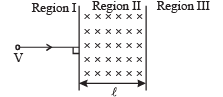Solution:

As the particle enters the magnetic field, a force acts on it due to the magnetic field which moves the particle in a circular path of radius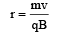For the particle to enter region III, r > l (path shown by dotted line)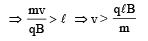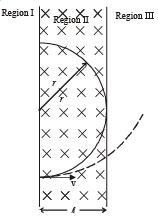For maximum path length in region  II, r = l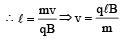The time taken by the particle to move in region II before coming back in region I is given by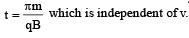*Multiple options can be correct
QUESTION: 15

Two metallic rings A and B, identical in shape and size but having different resistivities ρA and ρB, are kept on top of two identical solenoids as shown in the figure. When current I is switched on in both the solenoids in identical manner, the rings A and B jump to heights hA and hB, respectively, with hA > hB. The possible relation(s) between their resistivities and their masses mA and mB is(are)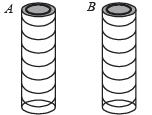Solution:

When current I is switched on in both the solenoids in identical manner, eddy currents are setup in metallic rings A and B in such a way that rings A and B are repelled.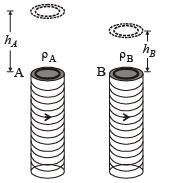Given hA >hB . This shows that eddy currents produced in A are greater than in B. This is possible when r A <rB (the rate of change of flux is same in both the rings, therefore induced emf is same).

*Multiple options can be correct
QUESTION: 16

An electron and a proton are moving on straight parallel paths with same velocity. They enter a semi infinite region of uniform magnetic field perpendicular to the velocity. Which of the following statement(s) is / are true?

Solution:

Figure shows that the megnetic field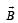is present on the right hand side of AB. The electron (e) and proton (p) moving on straight parallel paths with the same velocity enter the region of uniform magnetic field.
The entry and exit of electron & proton in the magnetic field makes the same angle with AB as shown.
Therefore both will come out travelling in parallel paths.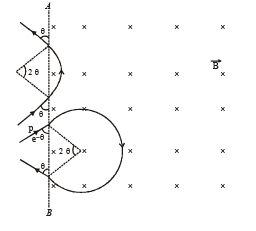The time taken by proton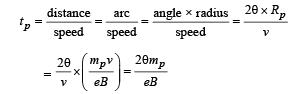The time taken by electron is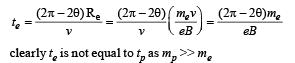∴ (b) , (d)  are correct options

*Multiple options can be correct
QUESTION: 17

Consider the motion of a positive point charge in a region where there are simultaneous uniform electric and magnetic fields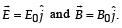At time t = 0, this charge has velocity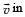the in the x-y plane, making an angle θ with the x-axis. Which of the following option(s) is (are) correct for time t > 0?

Solution:

When θ = 0º, the charged particle is projected along xaxis, due to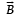the charged particle will tend to move in a circular path in y-z plane but due to force of electric field, the particle will move in a helical path with increasing pitch. Therefore options (A) and (B) are incorrect.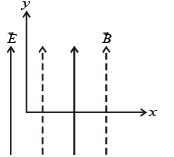When θ = 10º, we can resolve velocity into two rectangular components. One along x-axis (v cos 10º) and one along yaxis (v sin 10º). Due to v cos 10º, the particle will move in circular path and due to v sin 10º plus the force due to electric field, the particle will undergo helical motion with its pitch increasing.
If θ = 90º, the charge is moving along the magnetic field.
Therefore the force due to magnetic field is zero. But the force due to electric field will accelerate the particle along yaxis.

*Multiple options can be correct
QUESTION: 18

A particle of mass M and positive charge Q, moving with a constant velocity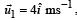enters a region of uniform static magnetic field, normal to the x-y plane. The region of the magnetic field extends from x = 0 to x = L for all values of y. After passing through this region, the particle emerges on the other side after 10 milliseconds with a velocity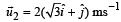. The correct statement(s) is (are)

Solution:

The magnetic field should be in the –z direction (Fleming’s left hand rule)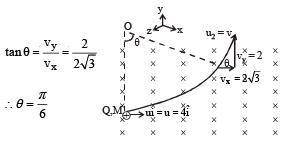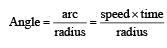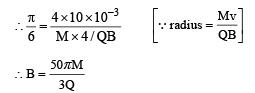(a) and (c) are the correct options

*Multiple options can be correct
QUESTION: 19

A steady current I flows along an infinitely long hollow cylindrical conductor of radius R. This cylinder is placed coaxially inside an infinite solenoid of radius 2R. The solenoid has n turns per unit length and carries a steady current I. Consider a point P at a distance r from the common axis. The correct statement(s) is (are)

Solution:

In the region O < r < R, the magnetic field is present due to current in solenoid.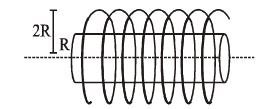In the region r > 2 R, the magnetic field is present due to the current in the cylinder.
For the region R < r < 2R, the magnetic field is neither along the common axis, nor tangential to the circle of radius r. (a) and (d) are correct options.

*Multiple options can be correct
QUESTION: 20

A conductor (shown in the figure) carrying constant current I is kept in the x-y plane in a uniform magnetic field r B . If F is the magnitude of the total magnetic force acting on the conductor, then the correct statement(s) is(are)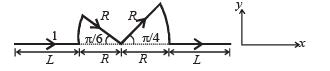Solution: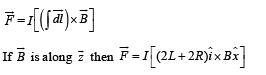option [A] is correct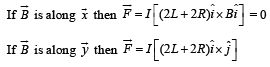Option (b) and (c) are also correct

*Multiple options can be correct
QUESTION: 21

Consider two identical galvanometers and two identical resistors with resistance R. If the internal resistance of the galvanometers RC < R/2, which of the following statement(s) about any one of the galvanometers is (are) true?

Solution:

The range of voltmeter ‘V’ is given by the expression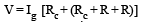V is max in this case as RHS is maximum. Thus (a) is correct.
The range of ammeter I is given by the expression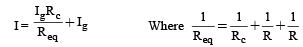Here Req is minimum and therefore I is maximum. Thus (c) is the correct option.